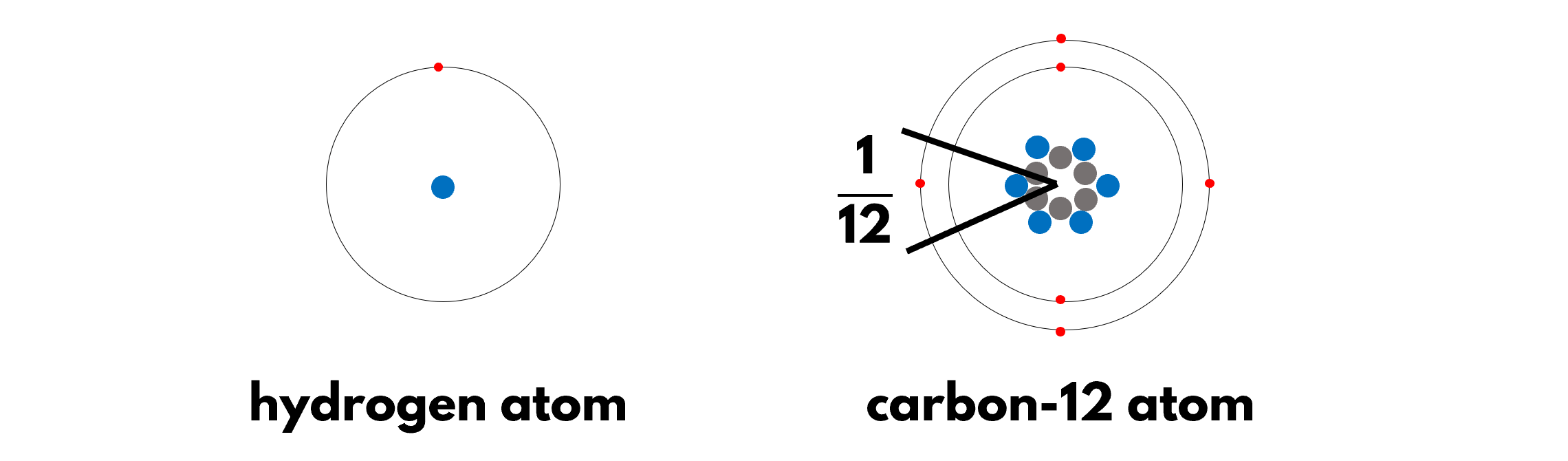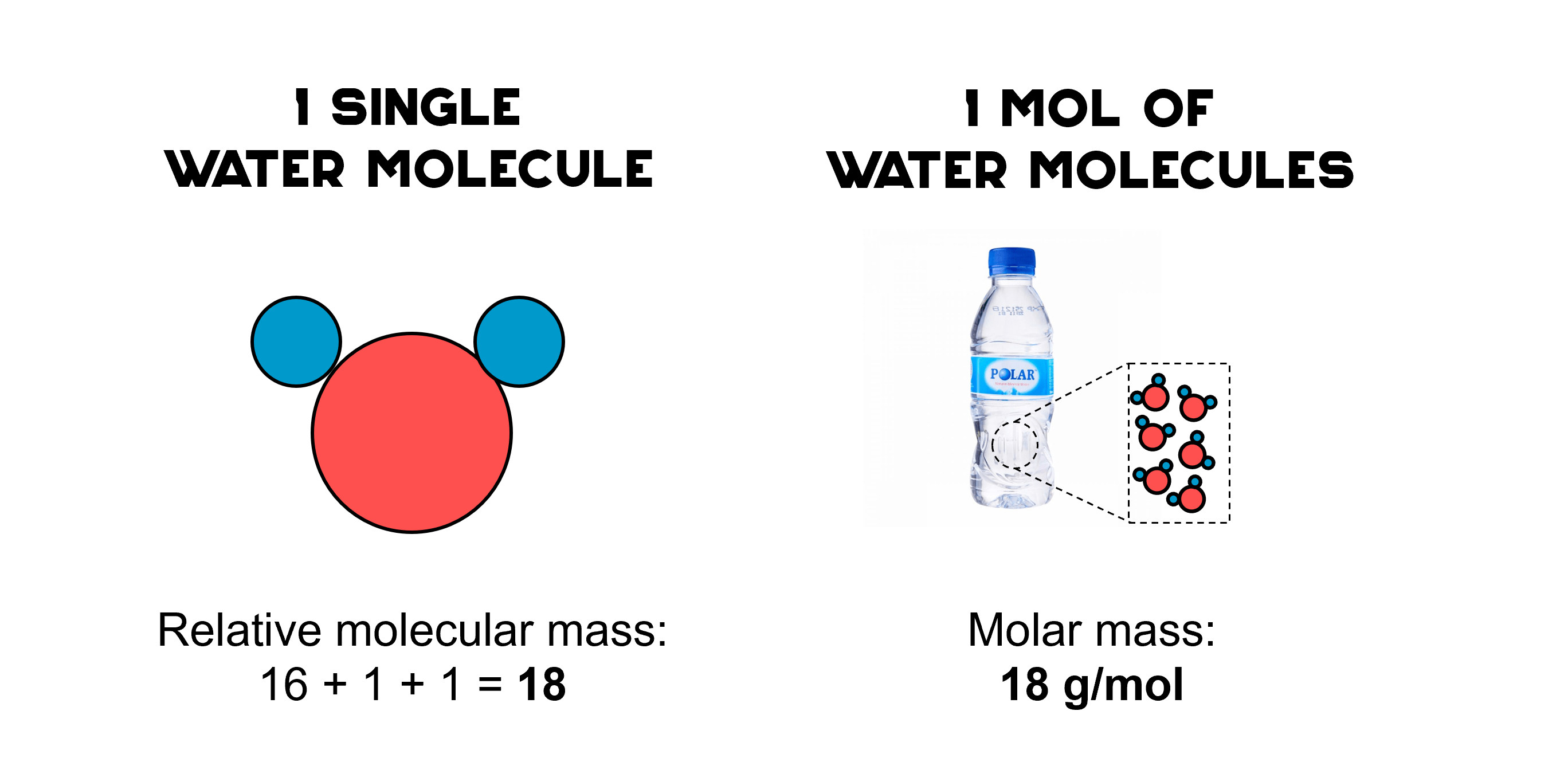## 1. Making sense of measurements with ratios

A cup of milk tea with pearls gives you 342 kcal of energy. What does this number mean? The paranoid will find it worrying, since it is a three-figure sum. The delusional BBT lovers will say that it’s less than a thousand and so it’s nothing much.

Alternatively, we can make sense of the number by thinking about it as a ratio. Comparing milk tea to a slice of bread, one cup gives you 5 slices’ worth of energy. On the other hand, a large packet of fries from McDonald’s gives you 7 slices’ worth of energy. Now I am worried.

## 2. Making sense of the mass of an atom with the ratio: relative atomic mass

The mass of an atom is so small that it is mind boggling to express it in grams. The mass of a hydrogen atom is about 1.67×10-30 g.

Instead, to describe the mass of a single atom, scientists use the relative atomic mass, which is a ratio. Like how we compared milk tea to bread slices, we compare an atom to a 1/12th “slice” of a carbon-12 atom. For example, a hydrogen atom has 1 slice’s worth of mass, a carbon atom 12 slices’ worth, and a magnesium atom 24 slices’ worth. Therefore, we say that hydrogen, carbon, and magnesium have a relative atomic mass of 1, 12, and 24 respectively.

Note that the relative atomic mass has no unit, as it is a ratio.

Relative atomic mass, Ar is defined as the ratio of the mass of an atom compared to 1/12th of the mass of an atom of carbon-12.

## 3. Relating relative atomic mass to the structure of an atomHydrogen has 1 proton (blue ball), while carbon-12 has 6 protons and 6 neutrons (grey balls). Electrons are represented by the small red balls.

The nucleus of carbon-12 has 12 nucleons, comprising 6 protons and 6 neutrons. Thus, 1/12th of its mass is about the mass of 1 nucleon. This is why hydrogen — with only 1 nucleon — has a relative atomic mass of 1.

Simply put, the relative atomic mass of an atom is equal to the number of nucleons it has.

## 4. The periodic table, your enabler

Good news! The relative atomic mass of every element is given in the periodic table. Just don’t mix up the proton (atomic) number with the relative atomic mass. Refer to the key to know which is which.

## 5. Relative atomic mass of an element: not your average kind of average

If you inspect your periodic table hard enough, you will realise that chlorine is an odd ball. It is the only element in the O-Level periodic table with a relative atomic mass that is not a whole number. This is because relative atomic mass is a sneaky concept. It is actually an average, though it does not explicitly tell you so.

In a sample of chlorine, most atoms exist as the chlorine-35 isotopes. However, a significant portion of 25% exist as the chlorine-37 isotopes. Therefore, the relative atomic mass of chlorine is the average of the relative molecular mass of all the chlorine atoms, be it chlorine-35 or chlorine-37.

In fact, for the other elements, their relative atomic mass only appears to be a whole number because they are rounded off to the nearest 0.5 in the O-Level periodic table. Referring to the A-Level periodic table, the relative atomic mass is more precise as it is rounded to the nearest 0.1. This shows that all elements have different isotopes. Their relative atomic mass is the weighted average of that of each isotope.

## 6. Relative molecular mass

A molecule is a chemical combination of atoms. In a similar vein, the relative molecular mass is simply the sum of the relative atomic mass of each of every atom in a molecule.

Relative molecular mass, Mr of XY = Ar of X + Ar of Y

Let’s try to find the relative molecular mass of a molecule of sucrose, C12H22O11.

• ##### STEP 1: State and interpret the chemical formula of the molecule

A molecule of C12H22O11 has 12 carbon atoms, 22 hydrogen atoms, 11 oxygen atoms.

• ##### STEP 2: Refer to the periodic table for the Ar of each constituent element

Ar of carbon = 12
Ar of hydrogen = 1
Ar of oxygen = 16

• ##### STEP 3: Sum the Ar of every atom in a molecule

Mr = 12×12 + 22×1 + 11×16 = 342

## 7. A relative of relative molecular mass: molar massThe relative molecular mass and molar mass of water have the same number, but different unit

The relative molecular mass of a single molecule is numerically equal to the mass of 1 mol of molecules in grams.

This means that when we put 6×1023 molecules of water (which is 1 mol of water) on a mass balance, the mass in grams will be exactly 18 g. This is the molar mass of water.